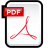Scientific journal NRU ITMO
Series "Processes and Food Production Equipment"
Registration certificate ЭЛ № ФС 77 – 55245 - 04.09.2013
registered by the Federal Inspectorate Service for Communication, Information Technologies and Communication Media
ISSN:2310-1164
Publications
Partners

December 2019 (published: 17.12.2019)

Number 4(42)

Home > Issue > Mathematical simulation of knife friction force during food cutting

## Mathematical simulation of knife friction force during food cutting

The process of friction when cutting food materials has been investigated theoretically. The muscle tissue of the raw material has been described by the Maxwell–Thomson rheological model. For choosing an analytical description of a regular microrelief of food processing equipment knives, taking into account technological formative factors, a physical-technological theory of surface roughness has been used. A mathematical description of the knife profile rough surface in the form of a periodic function with two harmonics has been considered. By solving the differential equation of viscoelastic material state, the law of distribution of contact pressure over the microprotrusions of the edge rough surface has been obtained. An expressions for dimension and dimensionless deformation friction forces has been obtained. It is established that at speeds tending to zero or infinity, this force tends to zero. The value of the dimensionless force increases monotonically with the increase in the measure of the material elasticity and the increase in the dimensionless length of the knife edge. The dimensionless width of the contact area of the irregularities monotonously depends on the measure of material elasticity and non-monotonously depends on the dimensionless sliding speed with a pronounced minimum. When the values of the elasticity measure is 5, and the dimensionless velocity is 0.001; 0.03; and 0.12, maximum contact pressure amplitude is 1.0; 1.391; and 2.750 respectively. With the values of elasticity measure of 2; 4; 7;and 12, minimum values of the dimensionless width of the contact pad are 0.683; 0.641; 0.612; and 0.588, the limit value is 0.684. With the values of the elasticity measure is 5; and dimensionless edge length is 10; 20; 30; and 50, the maximum values of the dimensionless deformation friction force are 21.728; 43.360; 64.993; and 108.258 respectively. When values of dimensionless edge length is 20; elasticity measure is 2; 5; 8; and 12, the values of the maximum force are 17.344; 43.360; 69.376; and 104.064 respectively.
Read the full articleKeywords: pretreatment of raw materials; food cutting; math modeling; friction force; knife roughness; rheology; viscoelasticity

### DOI 10.17586/2310-1164-2019-12-4-3-17